In the circuit given below, V(t) is the sinusoidal voltage source, voltage drop ${\mathrm{V}}_{\mathrm{AB}}$(t) across the resistance R is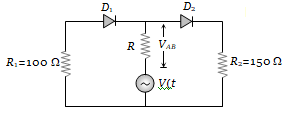(a) Is half wave rectified
(b) Is full wave rectified
(c) Has the same peak value in the positive and negative half cycles
(d) Has different peak values during positive and negative half cycle

Concept Questions :-

Applications of PN junction
High Yielding Test Series + Question Bank - NEET 2020

Difficulty Level:

The peak voltage in the output of a half-wave diode rectifier fed with a sinusoidal signal without filter is 10 V. The dc component of the output voltage is
(a)$10/\sqrt{2}$  V                                    (b) $10/\mathrm{\pi }$ V
(c) 10 V                                            (d) $20/\mathrm{\pi }$ V

Concept Questions :-

Rectifier
High Yielding Test Series + Question Bank - NEET 2020

Difficulty Level:

A transistor is used as an amplifier in CB mode with a load resistance of 5 k$\mathrm{\Omega }$. The current gain of the amplifier is 0.98 and the input resistance is 70 $\mathrm{\Omega }$, the voltage gain and power gain respectively are :
(a) 70, 68.6                          (b) 80, 75.6
(c) 60, 66.6                          (d) 90, 96.6

Concept Questions :-

Applications of Transistor
High Yielding Test Series + Question Bank - NEET 2020

Difficulty Level:

In the following circuits PN-junction diodes ${\mathrm{D}}_{1}$${\mathrm{D}}_{2}$ and ${\mathrm{D}}_{3}$ are ideal for the following potential of A and B, the correct increasing order of resistance between A and B will be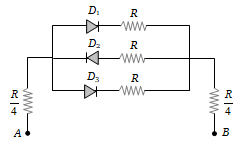(i) – 10 V, – 5V           (ii) – 5V, – 10 V
(iii) – 4V, – 12V

(a) (i) < (ii) < (iii)                       (b) (iii) < (ii) < (i)
(c) (ii) = (iii) < (i)                       (d) (i) = (iii) < (ii)

Concept Questions :-

Applications of PN junction
High Yielding Test Series + Question Bank - NEET 2020

Difficulty Level:

The circuit shown in the following figure contains two diode ${\mathrm{D}}_{1}$ and ${\mathrm{D}}_{2}$ each with a forward resistance of 50 ohms and with infinite backward resistance. If the battery voltage is 6 V, the current through the 100 ohm resistance (in amperes) is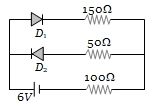(a) Zero                     (b) 0.02
(c) 0.03                     (d) 0.036

Concept Questions :-

PN junction
High Yielding Test Series + Question Bank - NEET 2020

Difficulty Level:

The contribution in the total current flowing through a semiconductor due to electrons and holes are $\frac{3}{4}$ and $\frac{1}{4}$ respectively. If the drift velocity of electrons is $\frac{5}{2}$ times that of holes at this temperature, then the ratio of concentration of electrons and holes is
(a) 6 : 5                 (b) 5 : 6
(c) 3 : 2                 (d) 2 : 3

Concept Questions :-

Types of semiconductors
High Yielding Test Series + Question Bank - NEET 2020

Difficulty Level:

Ge and Si diodes conduct at 0.3 V and 0.7 V respectively. In the following figure if Ge diode connection are reversed, the valve of ${\mathrm{V}}_{0}$ changes by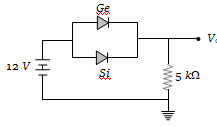(a) 0.2 V                    (b) 0.4 V
(c) 0.6 V                    (d) 0.8 V

Concept Questions :-

PN junction
High Yielding Test Series + Question Bank - NEET 2020

Difficulty Level:

In the circuit shown in the figure, the maximum output voltage ${\mathrm{V}}_{0}$ is :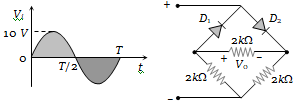1. 0 V                        2. 5 V
3. 10 V                      4. $\frac{5}{\sqrt{2}}$

Concept Questions :-

Rectifier
High Yielding Test Series + Question Bank - NEET 2020

Difficulty Level:

In the following circuit, find ${\mathrm{I}}_{1}$ and ${\mathrm{I}}_{2}$.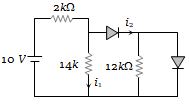(a) 0, 0
(b) 5 mA, 5 mA
(c) 5 mA, 0
(d) 0, 5 mA

Concept Questions :-

Applications of PN junction
High Yielding Test Series + Question Bank - NEET 2020

Difficulty Level:

For the transistor circuit shown below, if $\mathrm{\beta }$ = 100, voltage drop between emitter and base is 0.7 V, then value of ${\mathrm{V}}_{\mathrm{CE}}$ will be :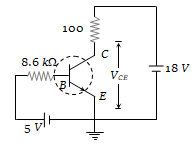(a) 10 V
(b) 5 V
(c) 13 V
(d) 0 V

Concept Questions :-

Transistor